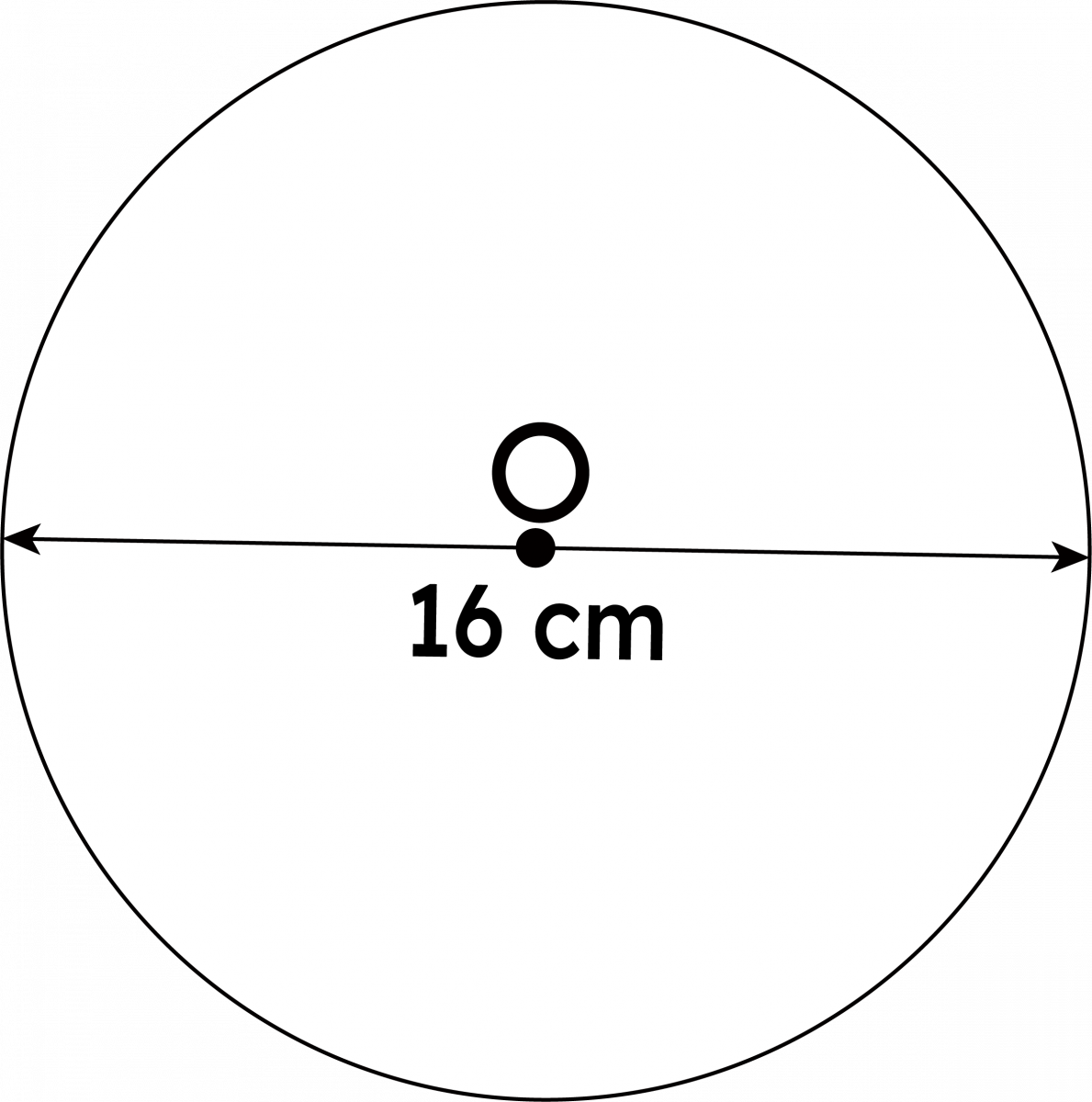# Value of Pi

The value of Pi (π) is defined as the ratio of the circumference of a circle to its diameter and equal to 3.14159 approximately. In a circle, if we divide the circumference (the total distance around the circle) by the diameter, you get the same number. Whether the circle is large or small, the value of pi remains the same. The symbol for Pi is denoted π and pronounced "pie." It is the 16 letters of the Greek alphabet and is used to represent a mathematical constant.

 Table of Content

## Pi Values in Fractions and Decimal

Pi (π) is mainly expressed in two different ways, which are:

• Value of pi(π) in fraction
• Value of pi(π) in decimal

### Value of Pi (π) in Decimal

The pi(π) value up to the 100 decimal places are- 3.14159 26535 89793 23846 26433 83279 50288 41971 69399 37510 58209 74944 59230 78164 06286 20899 86280 34825 34211 7067

### Value of Pi (π) in Fractions

The value of pi in the fraction is 22/7. It is known that a pi is an irrational number, which means that the digits after the decimal point are infinite and are a non-terminating value. Therefore, 22/7 is used for daily calculations. "π" is not equal to the ratio of any two numbers, making it an irrational number.

 All Values of Pi (π) In Decimal 3.14 In Fraction 22/7

### How to Calculate Value of Pi?

To calculate the value of π, the pi formula is used:

π = (Circumference/ Diameter)

Or, π = 3.14159 = 22/7

### Solved Examples

Q1. A man walks around a circle which has a diameter of 80 m, how far the man has walked?

Ans. Distance walked = Circumference.

Circumference = π × 80m = 251.2m

Therefore, distance walked =251.2m

Q2. Calculate the circumference of the circle.

Ans. As we know that the circumference of a circle is the distance around a circle.The Circumference formula is given by c = π × d.

Therefore, when substitute the value of d, we get;

C = π x 16

=3.14 x 16

= 50.24cm

Also Read For More- 1 Billion in RupeesNumber Names 1 to 100

Q1. What is pi used for?

Ans. In mathematics, pi calculates a circle's area and circumference. Pi can be used to find an area by multiplying the radius of the circle squared times pi.

Q2. Why is pi necessary?

Ans. Pi is necessary because it is a transcendental, irrational number – the digits that occur after the decimal point are called inexhaustible. They always go on forever and ever. The no. of digits is currently known to surpass 2 trillion! This implies that the number 3.14 or 3.145926 is an outrageous approximation.

Q3. What is PI in maths?

Ans. The number π is a mathematical constant that is the ratio of a circle's circumference to its diameter, approximately equal to 3.14159. The number π appears in many formulas across mathematics and physics.

Q4. What is the history of pi?

Ans. Oughtred, in 1647 used the symbol πd for the ratio of the diameter of a circle to its circumference. David Gregory (1697) used rπ for the ratio of the circumference of a circle to its radius. The first to use π with its present meaning was a Welsh mathematician William Jones in 1706 when he states, "3. 14159, etc.

Q5. Why is 3.14 called pi?

Ans. It was first known as "pi" in 1706 by a Welsh mathematician, i.e., William Jones, as π is the first letter in the greek word "perimitros," which means "perimeter."